# The Poisson Deviance for Regression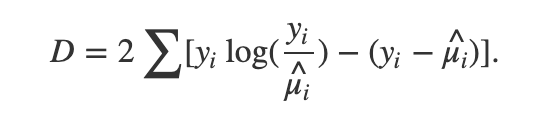Poisson Deviance

You’ve probably heard of the Poisson distribution, a probability distribution often used for modeling counts, that is, positive integer values. Imagine you’re modeling “events”, like the number of customers that walk into a store, or birds that land in a tree in a given hour. That’s what the Poisson is often used for. From the perspective of regression, we have this thing called Generalized Poisson Models (GPMs), where the response variable you are modeling is some kind of Poisson-ish type distribution. In a Poisson distribution, the mean is equal to the variance, but in real life, the variance is often greater (over-dispersed) or less than the mean (under-dispersed).

One of the most intuitive and easy to understand explanations of GPMs is from Sachin Date, who, if you don’t know, explains many difficult topics well. What I want to focus on here is something kind of related — the Poisson deviance, or the Poisson loss function.

Most of the time, your models, whether they are linear models, neural networks, or some tree-based method (XGBoost, Random Forest, etc.) are going to use mean squared error (MSE) or root mean squared error (RMSE) as your objective function. As you might know, MSE tends to favor the median, and RMSE and tends to favor the mean of a conditional distribution — this is why people worry about how the loss function works with the outliers in their data sets. However, most of the time, with some normally distributed response, you expect the values of the response (if z-score normalized, especially), to be some kind of Gaussian with mean 0 and unit variance. However, if you are dealing with count data — all positive integers — and you don’t scale or transform the response, then maybe a Poisson distribution is the better description of the data. When the mean of a Poisson is over 10, and especially over 20, it can be approximated with a Gaussian; the heavy tail that you see when the mean (the rate) is low tends to disappear.

Take a look at the formula in the beginning of the post — the y_i is the ground truth, and the mu_i is your model’s prediction. Obviously, if y_i = mu_i, then you have a ln(1), which is 0, canceling out the first term, and the second as well, giving a deviance of 0. What’s interesting is what happens when your model errs on either side of the actual value. In the following snippet, I plot the loss of one example where the ground truth is set at 20 — meaning that we assume the variable is Poisson distributed with mean/rate = 20.

`from matplotlib import pyplot as pltimport numpy as npxticks = np.linspace(start = 0.0000, stop = 50, num = int(1e4))yi = 20losses = [2 * (yi * np.log(yi / x) - (yi - x)) for x in xticks]losses = np.array(losses)plt.scatter(xticks, losses)`

Here’s the graph: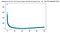Poisson deviance as a function of model output. The rate/ground truth is set at 20 in this example, which is the minimum of the points you see here.

So what does this tell you? Well, if your model guesses between 10 and even 50, it doesn’t look like the deviance is that high — at least not compared with what happens if you guess 1 or 2. This is obviously a lot different from other loss functions: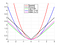Some typical loss functions.

So if your model outputs a 0 when the ground truth as 20, then, if you’re using MSE, the loss is 20² = 400, whereas, for the Poisson deviance, you would get infinite deviance, which is, uh, not good. The model isn’t supposed to really output 0, since there’s really not much probability mass there. If your model outputted something like .0001, your loss would be in the ballpark of >400. If your model outputs 30, and the ground truth was 20, your loss would only be around 3.78, where was in MSE terms, it would be 10² = 100.

So the moral of the story is that yes, the model should favor the mean of the distribution, but using Poisson deviance means you won’t penalize it that heavily for being biased ABOVE the mean — like if your model kept outputting 30 or 35, even if the distribution’s mean is 20. But your model will be penalized very heavily for outputting anything between 0 and 10. Why?

Well, what is the (log)likelihood function of the Poisson distribution?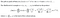Poisson likelihood function

Now if you want to look at something a little more tractable, take the log of that —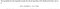Poisson Log-Likelihood function

If your model keeps outputting 0, then t = 0, and that t * ln(lambda) expression is going to be 0, leaving you with a large negative number from the first term. In fact, the only way that your LL can even be close to “good” is if t — the sum of the observations — is sufficiently large for the second term to counteract the first term. That’s why, I believe, low values between 0–10 (again, assuming the rate/mean is 20) are penalized so heavily.

OK, so let’s say you’re sufficiently interested in the Poisson deviance. How can you calculate it? Well, from the perspective of the Python user, you have sklearn.metrics.mean_poisson_deviance — which is what I have been using. And you have Poisson loss as a choice of objective function for all the major GBDT methods — XGBoost, LightGBM, CatBoost, and HistGradientBoostingRegressor in sklearn. You also have PoissonRegressor() in the 0.24 release of sklearn…in any case, there are many ways you can incorporate Poisson type loss into training.

Does it help, though? Tune in for my next project, where I will see just how these Poisson options play out on real life data sets.

Machine Learning Engineer

## More from Peijin Chen

Machine Learning Engineer